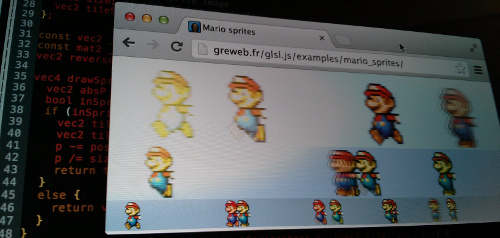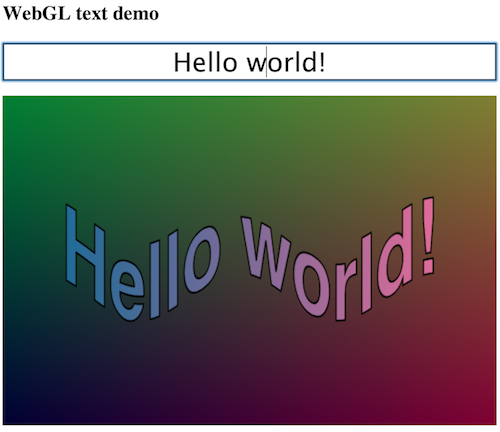# glsl.js, a Javascript + GLSL library = DRY & efficientTL;DR. WebGL is super powerful and efficient. This library abuses this power for efficient 2D.

glsl.js is a subset of a WebGL library which focuses on making the GLSL (OpenGL Shading Language) easy and accessible for vizualisation and game purposes (2D or 3D).## Why?

WebGL is a very low level and stateful API. Actually the WebGL API is the OpenGL API.

Do you know glsl.heroku.com? It’s a cool platform for demoscene where you can experiment some nice effects in GLSL. My library extends this concept of rendering in one whole fragment shader (which takes the plain canvas) but also provides a way to inject your own Javascript variables.

### DRY

WebGL is not DRY at all, you always have to repeat yourself both on the GLSL and on the Javascript part (especially for synchronizing variables).
Worse than that, you have to know in your Javascript code what are the GLSL types of every variable to synchronize.

How boring is that:

``````// Synchronizing the new values of 2 variables in pure WebGL.

var myInt = 1;
var myIntLocation = gl.getUniformLocation(program, "myInt");
myInt;
gl.uniform1i(myFloatLocation, myInt); // 1i means one integer

var myVector2 = { x: 1.3, y: 2.4 };
var myVector2Location = gl.getUniformLocation(program, "myVector2");
gl.uniform2f(myVector2Location, myVector2.x, myVector2.y); // 2f means float
``````

glsl.js provides a DRY and simple way to synchronize Javascript variables.

First, the library will handle for you the UniformLocations.

More important, and unlike the WebGL API and many WebGL libraries, you will never have to define the type of your variables from the Javascript with glsl.js! You just define it once in your shader!

How it works behind is the framework will statically parse your GLSL and infer types to use for the synchronization. The right `gl.uniform*` function is called by Javascript reflection.

It now simply becomes:

``````// Set the values of 2 variables in glsl.js
this.set("myInt", 1);
this.set("myVector2", { x: 1.3, y: 2.4 });
``````

More technically, glsl.js is a subset* of a WebGL library which focus on making the GLSL (OpenGL Shading Language) easy and accessible for vizualisation and game purposes (2D or 3D).

* Subset, because we only focus on using a fragment shader (the vertex shader is static and take the full canvas size), But don’t worry, you have a long way to go with just one fragment shader.

The concept is to split the rendering part in a GLSL fragment from the logic part in Javascript of your app/game. Both part are linked by a set of variables (the state of your app/game).glsl.js aims to abstract every GL functions so you don’t have to learn any OpenGL API.
What you only need to care about is the logic in Javascript and the rendering in GLSL.

By design, you can’t mix logic and render part, this approach really helps to focus on essential things separately.

### Efficiency

Basically, this design is efficient because the Javascript logic will take some CPU while the rendering will take the graphic card.

Today, WebGL is widely supported on modern desktop browsers. It’s not yet the case on mobile and tablet.

However, using Chrome Beta, I’m able to run my HTML5 game at 60fps on my Nexus 4, which is quite promising for the future.

Enough talking, let’s see some examples now…

### Hello World Example

Here is an Hello World example. For more examples, see /examples.

``````<canvas id="viewport" width="600" height="400"></canvas>
#ifdef GL_ES
precision mediump float;
#endif
uniform float time;
uniform vec2 resolution;
void main (void) {
vec2 p = ( gl_FragCoord.xy / resolution.xy );
gl_FragColor = vec4(p.x, p.y, (1.+cos(time))/2., 1.0);
}
</script>
<script src="../../glsl.js" type="text/javascript"></script>
<script type="text/javascript">
var glsl = Glsl({
canvas: document.getElementById("viewport"),
fragment: document.getElementById("fragment").textContent,
variables: {
time: 0, // The time in ms
},
update: function (time, delta) {
this.set("time", time);
},
}).start();
</script>
``````GLSL is a high-level shading language based on the syntax of the C programming language. (Wikipedia)

GLSL gives a very different way of thinking the rendering: basically, in a main function, you have to set the color (`gl_FragColor`) of a pixel for a given position (`gl_FragCoord`).

As a nice side effect, GLSL is vectorial by design: it can be stretch to any dimension.

GLSL is efficient because it is compiled to the graphic card.

GLSL provides an interesting collection of types (e.g. `int`, `float`, `vec2`, `vec3`, `mat3`, `sampler2D`,… and also arrays of these types) and functions (e.g. `cos`, `smoothstep`, …).

You can also deeply explore the awesome collection of glsl.heroku.com. Any of glsl.heroku.com examples are compatible with glsl.js if you add some required variables (*time*, mouse, …).

### App/Game Logic

You must give to Glsl a `canvas` (DOM element of a canvas), a `fragment` (the GLSL fragment code), the `variables` set, and the `update` function.

Then you can start/stop the rendering via method (`.start()` and `.stop()`).

The `update` function is called as soon as possible by the library. It is called in a `requestAnimationFrame` context.

You must define all variables shared by both logic and render part in a Javascript object `{varname: value}`.
Variables must match your GLSL uniform variables. Every time you update your variables and you want to synchronize them with the GLSL you have to manually call the `sync` function by giving all variables name to synchronize.

Exemple:

``````Glsl({
canvas: canvas,
fragment: fragCode,
variables: {
time: , // The time in seconds
random1:
},
update: function (time, delta) {
this.set("time", time);
this.set("random1", Math.random());
}
}).start();
``````

Note: under the hood, a type environment of uniform variables is inferred by parsing your GLSL code.

### Using arrays

Hopefully, GLSL also supports arrays. You can actually bind a Javascript array to a GLSL uniform variable.

Example:

In GLSL,

``````uniform float tenfloats;
``````

In Javascript,

``````var glsl = Glsl({
...
variable: {
tenfloats: new Float32Array(10)
},
update: function () {
this.tenfloats = Math.random();
this.sync("tenfloats");
}
}).start();
``````

Alternatively, you can still use a classical javascript Array (but native Javascript arrays are prefered because more efficient).

Use `Int32Array` for `int[]` and `bool[]`.

Vector arrays are also possible. In Javascript, you will have to give a linearized array.
For instance,
a `vec2` will be `[vec2(1.0, 2.0), vec2(3.0, 4.0)]` if `Float32Array(1.0, 2.0, 3.0, 4.0)` is used.

### Using objects

Even more interesting now, you can synchronize a whole object into the GLSL world. This is very interesting for Object-Oriented approach.

Example:

In GLSL,

``````struct Circle {
vec2 center;
}
uniform Circle c1;
bool inCircle (vec2 p, Circle c) {
vec2 ratio = resolution/resolution.x;
}
void main (void) {
vec2 p = ( gl_FragCoord.xy / resolution.xy );
if (inCircle(p, c1))
gl_FragColor = vec4(1.0, 0.0, 0.0, 1.0);
else
gl_FragColor = vec4(0.0, 0.0, 0.0, 1.0);
}
``````

In Javascript,

``````function Circle (x, y, radius) {
this.center = { x: x, y: y };
}
Circle.prototype.update = function () {
}
var c1 = new Circle(0.5, 0.5, 0.1);
Glsl({
...
variable: {
c1: c1
},
update: function (time, delta) {
c1.update();
this.sync("c1");
}
}).start();
``````

structs inside structs are also supported:

``````struct Circle {
vec2 center;
}
struct Player {
Circle circle;
bool visible;
}
``````

### Using Arrays of Objects

The two previous chapters can be assemble!

Yes man, Array of JS object is possible!

``````uniform Circle circles;
// …
``````
``````Glsl({
...
variable: {
circles: [ new Circle(0.1, 0.1, 0.2), new Circle(0.2, 0.3, 0.2) ]
},
...
}).start();
``````

### Using images

GLSL:

``````uniform sampler2D img;
``````

Javascript:

``````var image = new Image();
img.src = "foo.png";
var glsl = Glsl({
...
variable: {
img: image
}
});
glsl.start();
}
``````

Note: Using an image loader library can be a good idea.

In GLSL, you will need to use the texture lookup functions to access the image color for a given coordinate. E.g. `texture2D(img, coord)`. (see the specs).

The mario_sprites example

### Using another canvas### Using